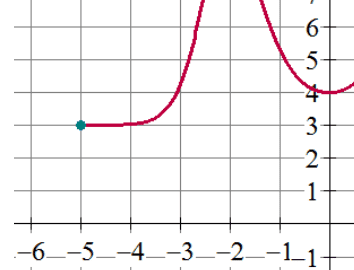# Limit on the edge of the domain

curious_ocean
Homework Statement:
What is the limit as x--> -5?
Relevant Equations:
What is the limit on the edge of a function's domain?
What is the limit of the function as x goes to -5 (e.g. in the graph below) if the domain of the function is only defined on the closed interval [-5,5]?
I realize that the right hand limit DOES exist and is equal to 3, but the left hand limit does not exist?
So does that mean that the overall limit does not exist?
Or since the domain does not exist to the left, is the left hand limit not needed for the overall limit?#### Attachments

Mentor
Homework Statement:: What is the limit as x--> -5?
Relevant Equations:: What is the limit on the edge of a function's domain?

What is the limit of the function as x goes to -5 (e.g. in the graph below) if the domain of the function is only defined on the closed interval [-5,5]?
I realize that the right hand limit DOES exist and is equal to 3, but the left hand limit does not exist?
Right. Only the right-hand limit exists at the left endpoint of the domain.
curious_ocean said:
So does that mean that the overall limit does not exist?
Right. For the limit to exist, both one-sided limits have to exist and be equal to each other.
curious_ocean said:
Or since the domain does not exist to the left, is the left hand limit not needed for the overall limit?
View attachment 323428

Homework Helper
Gold Member
2022 Award
Right. For the limit to exist, both one-sided limits have to exist and be equal to each other.
I don't agree with this. If you apply the definition of the limit to that function, then the limit exists. The need for left hand and right hand limits to exist does not apply at an endpoint of the domain.

Or since the domain does not exist to the left, is the left hand limit not needed for the overall limit?
That's what I would say.

•SammyS and pasmith
Mentor
If you apply the definition of the limit to that function, then the limit exists.
It depends on what definition you use, and whether you specify that only points in the domain can be used in the ##\delta-\epsilon## definition.

Homework Helper
2022 Award
It depends on what definition you use, and whether you specify that only points in the domain can be used in the ##\delta-\epsilon## definition.

You can't evaluate a function at points outside its domain. This is basic set theory.

There are three quantifiers in the formal definition of $\lim_{x \to a} f(x) = L$: $$(\forall \epsilon > 0)(\exists \delta > 0)(\forall x)(|x - a| < \delta \Rightarrow |f(x) - L| < \epsilon)).$$ The set over which $x$ is quantified must be some subset of the domain, or else we can't evaluate $f(x)$.

If you are given a domain which is an interval, then as far as you are concerned nothing outside of the closure of that interval exists. So the limit at an end point is necessarily the appropriate one-sided limit.

•PeroK
Homework Helper
I would suggest that to be clear, the formal definition above should contain explicitly the statement that x is in the domain of f as a hypothesis of the implication, in addition to the hypothesis that |x-a|<d. I.e. it should read that "if x is in the domain and |x-a| < d, then.." This would render the explanatory next- to-last sentence unnecessary, and then the last sentence would be a logical consequence.

Homework Helper
2022 Award
I would suggest that to be clear, the formal definition above should contain explicitly the statement that x is in the domain of f as a hypothesis of the implication, in addition to the hypothesis that |x-a|<d. I.e. it should read that "if x is in the domain and |x-a| < d, then.." This would render the explanatory next- to-last sentence unnecessary, and then the last sentence would be a logical consequence.

But $x$ must still be quantified over some set, and adding the condition that $x$ is in the domain to the premise of the implication adds nothing to the requirement that the set over which it is quantified must be a subset of the domain (and have $a$ as a limit point).

Staff Emeritus
Gold Member
Let's put this concept to the test.

##f(x)=\mathbb{1}_{\mathbb{Q}}## is the indicator function of the rational- 1 if x is rational, 0 otherwise.

Is ##1/f(x)## continuous?

Mentor
I would suggest that to be clear, the formal definition above should contain explicitly the statement that x is in the domain of f as a hypothesis of the implication, in addition to the hypothesis that |x-a|<d. I.e. it should read that "if x is in the domain and |x-a| < d, then.." This would render the explanatory next- to-last sentence unnecessary, and then the last sentence would be a logical consequence.
This was my point in post #4, in which I said it depended on which definition you used.